Ge and Si diodes conduct at 0.3 V and 0.7 V respectively. In the following figure if Ge diode connection are reversed, the valve of ${\mathrm{V}}_{0}$ changes by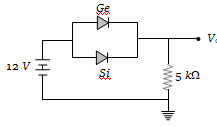(a) 0.2 V                    (b) 0.4 V
(c) 0.6 V                    (d) 0.8 V

Concept Questions :-

PN junction
High Yielding Test Series + Question Bank - NEET 2020

Difficulty Level:

In the circuit shown in the figure, the maximum output voltage ${\mathrm{V}}_{0}$ is :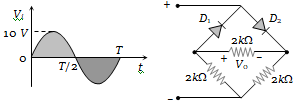1. 0 V                        2. 5 V
3. 10 V                      4. $\frac{5}{\sqrt{2}}$

Concept Questions :-

Rectifier
High Yielding Test Series + Question Bank - NEET 2020

Difficulty Level:

In the following circuit, find ${\mathrm{I}}_{1}$ and ${\mathrm{I}}_{2}$.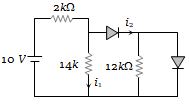(a) 0, 0
(b) 5 mA, 5 mA
(c) 5 mA, 0
(d) 0, 5 mA

Concept Questions :-

Applications of PN junction
High Yielding Test Series + Question Bank - NEET 2020

Difficulty Level:

For the transistor circuit shown below, if $\mathrm{\beta }$ = 100, voltage drop between emitter and base is 0.7 V, then value of ${\mathrm{V}}_{\mathrm{CE}}$ will be :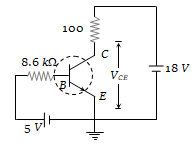(a) 10 V
(b) 5 V
(c) 13 V
(d) 0 V

Concept Questions :-

Transistor
High Yielding Test Series + Question Bank - NEET 2020

Difficulty Level:

The following configuration of gate is equivalent to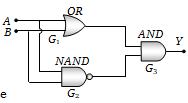(a) NAND
(b) XOR
(c) OR
(d) None of these

Concept Questions :-

Logic gates
High Yielding Test Series + Question Bank - NEET 2020

Difficulty Level:

Figure gives a system of logic gates. From the study of truth table it can be found that to produce a high output (1) at R, we must have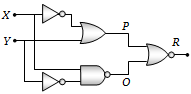(a) X = 0, Y = 1
(b) X = 1, Y = 1
(c) X = 1, Y = 0
(d) X = 0, Y = 0

Concept Questions :-

Logic gates
High Yielding Test Series + Question Bank - NEET 2020

Difficulty Level:

The combination of gates shown below produces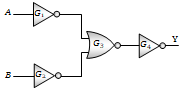(a) AND gate
(b) XOR gate
(c) NOR gate
(d) NAND gate

Concept Questions :-

Logic gates
High Yielding Test Series + Question Bank - NEET 2020

Difficulty Level:

The shows two NAND gates followed by a NOR gate. The system is equivalent to the following logic gate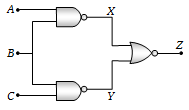(a) OR
(b) AND
(c) NAND
(d) None of these

Concept Questions :-

Logic gates
High Yielding Test Series + Question Bank - NEET 2020

Difficulty Level:

The diagram of a logic circuit is given below. The output F of the circuit is represented by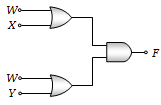(a) W(X+Y)
(b) W.(X.Y)
(c) W+(X.Y)
(d) W+(X+Y)

Concept Questions :-

Logic gates
High Yielding Test Series + Question Bank - NEET 2020

Difficulty Level:

The contribution in the total current flowing through a semiconductor due to electrons and holes are $\frac{3}{4}$ and $\frac{1}{4}$ respectively. If the drift velocity of electrons is $\frac{5}{2}$ times that of holes at this temperature, then the ratio of concentration of electrons and holes is
(a) 6 : 5                 (b) 5 : 6
(c) 3 : 2                 (d) 2 : 3

Concept Questions :-

Types of semiconductors